RGB LCD 示例

# 先介绍时序

RGB LCD 显示图像的原理和 VGA 类似，都是在计算机内部以数字的方式生成需要显示的图像信息，再通过模数转换的方式，将这些数字的图像信息转变为 RGB 三原色模拟信号，以及行、场同步信号。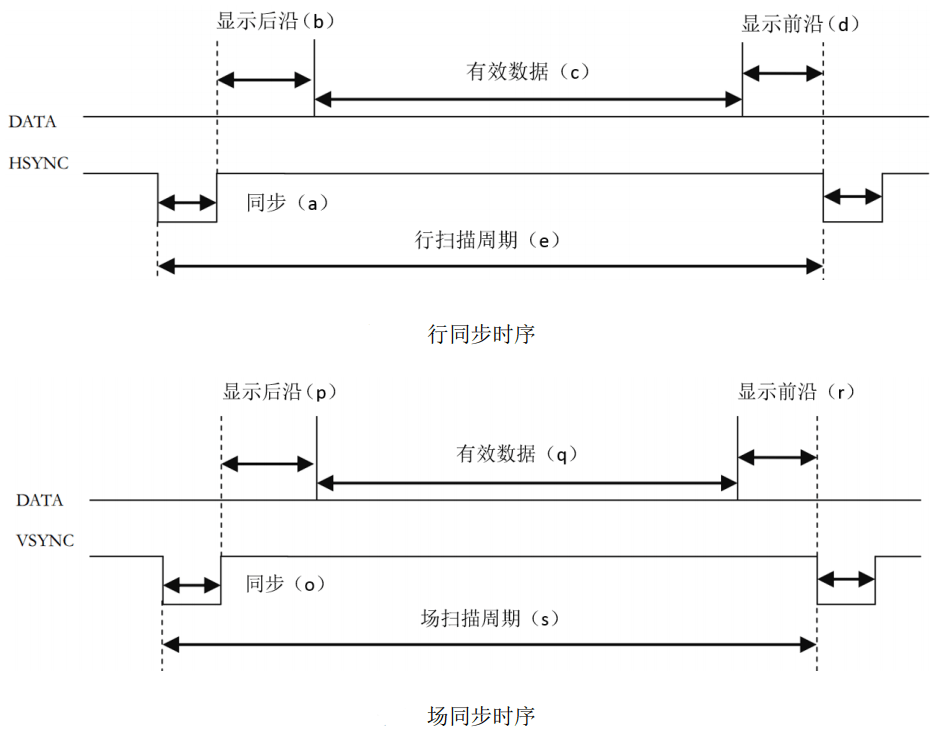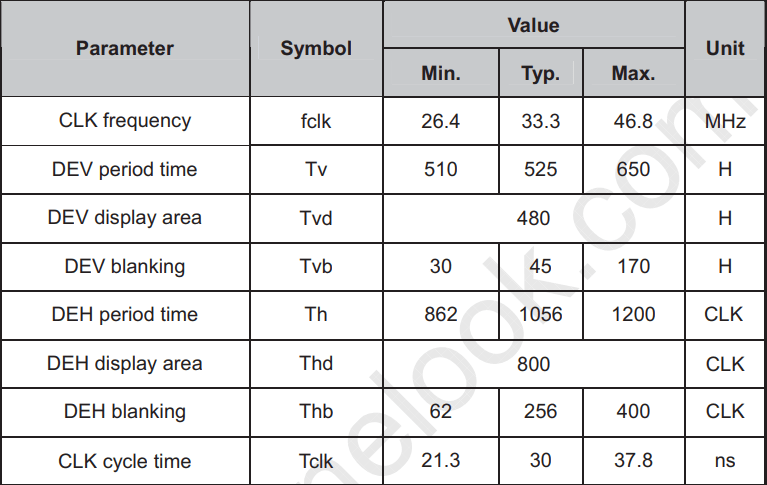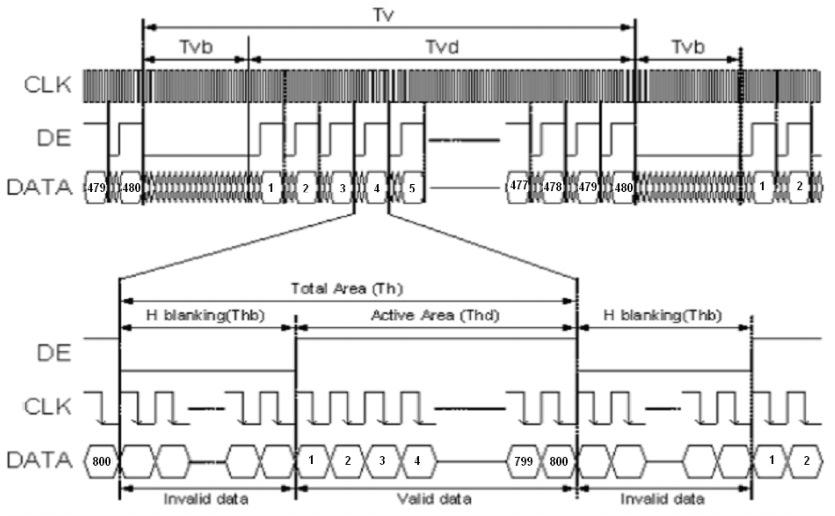# Verilog实现

## pll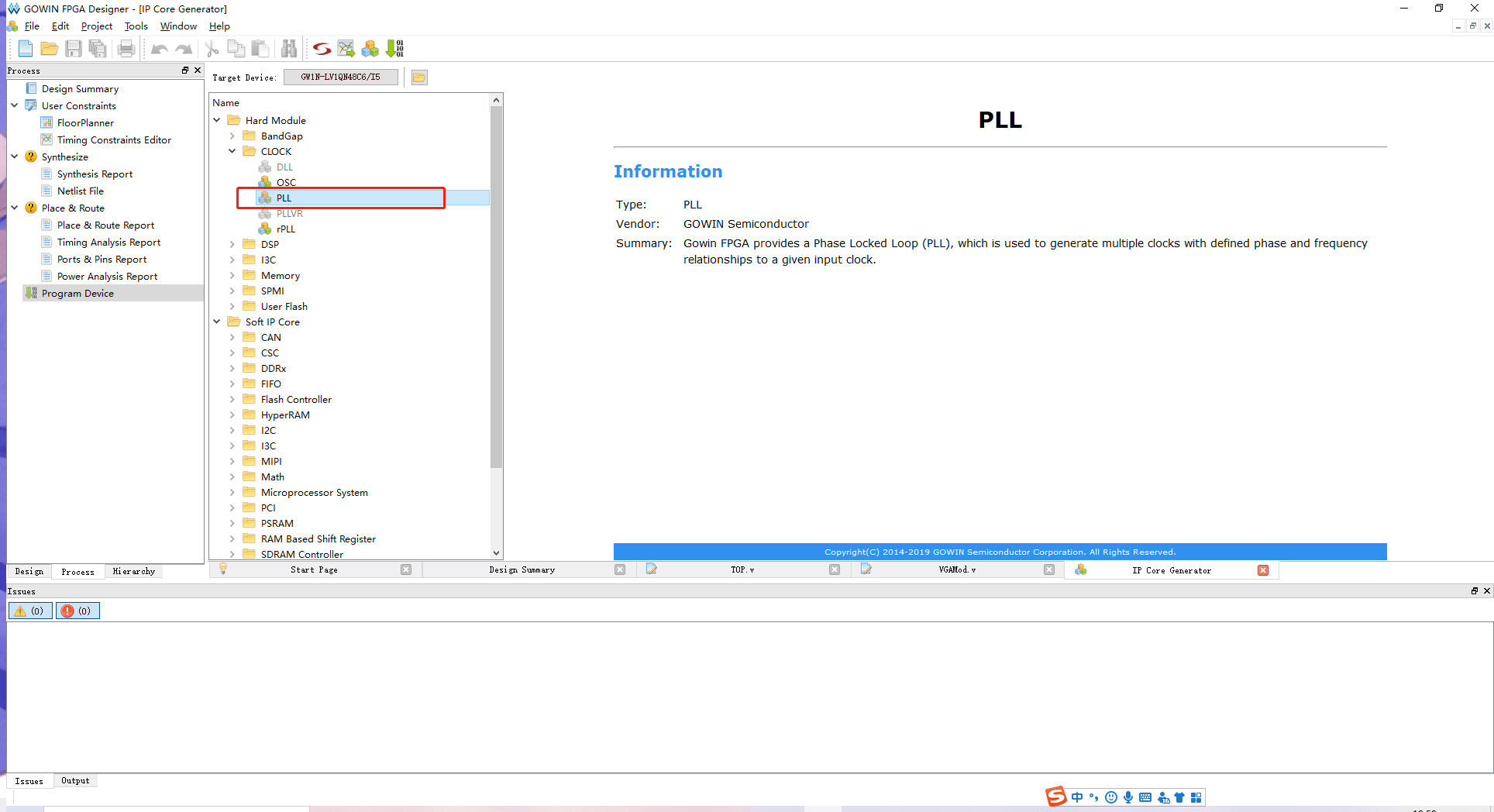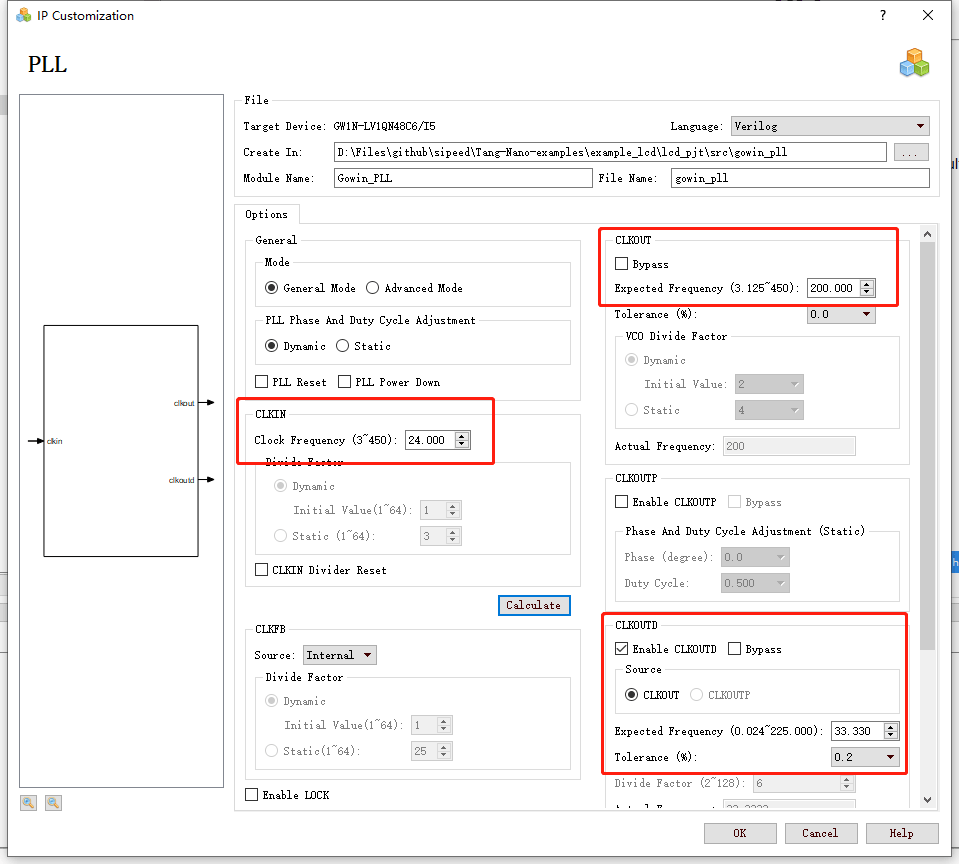## lcd时序产生

localparam      V_BackPorch = 16'd6; //0 or 45
localparam      V_Pluse 	= 16'd5;
localparam      HightPixel  = 16'd480;
localparam      V_FrontPorch= 16'd62; //45 or 0

localparam      H_BackPorch = 16'd182; 	//NOTE: 高像素时钟时，增加这里的延迟，方便K210加入中断
localparam      H_Pluse 	= 16'd1;
localparam      WidthPixel  = 16'd800;
localparam      H_FrontPorch= 16'd210;

localparam      PixelForHS  =   WidthPixel + H_BackPorch + H_FrontPorch;
localparam      LineForVS   =   HightPixel + V_BackPorch + V_FrontPorch;


always @(  posedge PixelClk or negedge nRST  )begin
if( !nRST ) begin
LineCount       <=  16'b0;
PixelCount      <=  16'b0;
end
else if(  PixelCount  ==  PixelForHS ) begin
PixelCount      <=  16'b0;
LineCount       <=  LineCount + 1'b1;
end
else if(  LineCount  == LineForVS  ) begin
LineCount       <=  16'b0;
PixelCount      <=  16'b0;
end
end

//注意这里HSYNC和VSYNC负极性
assign  LCD_HSYNC = (( PixelCount >= H_Pluse)&&( PixelCount <= (PixelForHS-H_FrontPorch))) ? 1'b0 : 1'b1;
assign  LCD_VSYNC = ((( LineCount  >= V_Pluse )&&( LineCount  <= (LineForVS-0) )) ) ? 1'b0 : 1'b1;


assign  LCD_DE = (  ( PixelCount >= H_BackPorch )&&
( PixelCount <= PixelForHS-H_FrontPorch ) &&
( LineCount >= V_BackPorch ) &&
( LineCount <= LineForVS-V_FrontPorch-1 ))  ? 1'b1 : 1'b0;
//这里不减一，会抖动


    localparam          Colorbar_width   =   WidthPixel / 16;

assign  LCD_R     = ( PixelCount < ( H_BackPorch +  Colorbar_width * 0  )) ? 5'b00000 :
( PixelCount < ( H_BackPorch +  Colorbar_width * 1  )) ? 5'b00001 :
( PixelCount < ( H_BackPorch +  Colorbar_width * 2  )) ? 5'b00010 :
( PixelCount < ( H_BackPorch +  Colorbar_width * 3  )) ? 5'b00100 :
( PixelCount < ( H_BackPorch +  Colorbar_width * 4  )) ? 5'b01000 :
( PixelCount < ( H_BackPorch +  Colorbar_width * 5  )) ? 5'b10000 :  5'b00000;

assign  LCD_G    =  ( PixelCount < ( H_BackPorch +  Colorbar_width * 6  )) ? 6'b000001:
( PixelCount < ( H_BackPorch +  Colorbar_width * 7  )) ? 6'b000010:
( PixelCount < ( H_BackPorch +  Colorbar_width * 8  )) ? 6'b000100:
( PixelCount < ( H_BackPorch +  Colorbar_width * 9  )) ? 6'b001000:
( PixelCount < ( H_BackPorch +  Colorbar_width * 10 )) ? 6'b010000:
( PixelCount < ( H_BackPorch +  Colorbar_width * 11 )) ? 6'b100000:  6'b000000;

assign  LCD_B    =  ( PixelCount < ( H_BackPorch +  Colorbar_width * 12 )) ? 5'b00001 :
( PixelCount < ( H_BackPorch +  Colorbar_width * 13 )) ? 5'b00010 :
( PixelCount < ( H_BackPorch +  Colorbar_width * 14 )) ? 5'b00100 :
( PixelCount < ( H_BackPorch +  Colorbar_width * 15 )) ? 5'b01000 :
( PixelCount < ( H_BackPorch +  Colorbar_width * 16 )) ? 5'b10000 :  5'b00000;


VGAMod	D1
(
.CLK		(	CLK_SYS     ),
.nRST		(	nRST		),

.PixelClk	(	CLK_PIX		),
.LCD_DE		(	LCD_DEN	 	),
.LCD_HSYNC	(	LCD_HYNC 	),
.LCD_VSYNC	(	LCD_SYNC 	),

.LCD_B		(	LCD_B		),
.LCD_G		(	LCD_G		),
.LCD_R		(	LCD_R		)
);